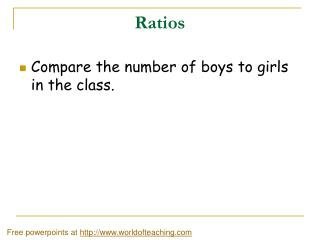DownloadDownload PresentationRatios

# Ratios

Télécharger la présentation## Ratios

- - - - - - - - - - - - - - - - - - - - - - - - - - - E N D - - - - - - - - - - - - - - - - - - - - - - - - - - -
##### Presentation Transcript

1. Ratios • Compare the number of boys to girls in the class. Free powerpoints at http://www.worldofteaching.com

2. The number of boys = • The number of girls = • If we compare boys to girls we get ___ boys to _____ girls.

3. What do we call a comparison between two or more quantities? We just found the RATIO of boys to girls. RATIO Is the ratio of girls to boys the same ? No, when writing a ratio, ORDER matters.

4. AIM: What is a ratio?

5. It’s Friday night and your friends are having a party…… The ratio of girls to guys is 2 to 12. Would you want to attend the party?

6. How many basketballs to footballs are there? • For every 4 basketballs there are 6 footballs. • The ratio is 4 to 6.

7. What are some other ways we can write the ratio of basketball to footballs? • 4 to 6 • 4 : 6 • 4 6 Every ratio can be written in 3 ways: First quantity to Second quantity Careful!! Order matters in a ratio. 4 to 6 Is NOT the same as 6 to 4 First quantity : Second quantity First quantity divided by the second quantity (as a fraction).

8. Write the ratio of sandwiches to coke bottles 3 different ways. • 6:8 , 6 to 8, and 6 8 Since a fraction can be simplified, We can simplify the ratio 6/8 to 3/4. The ratio of sandwiches to coke bottles can also be expressed as 3 : 4 or 3 to 4. In other words, ratios can be simplified to form equivalent ratios.

9. Equivalent Ratios • = 4 / 4 = 1 = 1 to 2 • 8 8 / 4 2 • Simplify the following ratios: • 4 to 8 • 10 to 8 • 8 to 10 GCF = 4 Step 1 – Write the ratio as a fraction Step 2 – Simplify the fraction (Find the greatest common factor (GCF) of both numbers and divide the numerator and denominator by the GCF). Step 3 – Write the equivalent ratio in the same form as the question

10. Equivalent Ratios can be formed by multiplying the ratio by any number. • For example, the ratio 2 : 3 can also be written as • 4 : 6 (multiply original ratio by by 2) • 6 : 9 (multiply original ratio by by 3) • 8 : 12 (multiply original ratio by by 4) • The ratio 2 : 3 can be expressed as • 2x to 3x (multiply the original ratio by any number x)

11. Compound Ratios • A ratio that compares more than 2 quantities is called a compound ratio. • Example: • A cake recipe says the ratio of cups of milk, sugar, and batter are 1:2:4. • This means that there is one cup of milk for every two cups of sugar and four cups of batter.

12. A bag contains 18 yellow, blue, and red marbles. The ratio of yellow to blue to red marbles is 4 : 2 : 3. • Write the ratio of yellow to blue marbles in simplest form. • What is the ratio of yellow to red marbles? 4 : 2 can be simplified to 2 : 1 4 : 3

13. Practice problem # 1 (1) You have 100 different shirts. The ratio of blue to black shirts is 20 . 30 a) Write the ratio of blue to black shirts 3 different ways. b) Write the ratio in simplest form. c) Explain what this ratio tells us. d) How many black shirts do you have?

14. Solution - # 1  You have 100 different shirts. The ratio of blue to black shirts is 20 / 30 a) Write the ratio of blue to black shirts 3 different ways. 20 to 30 , 20 : 30, 20 30 b) Write the ratio in simplest form.2 3 c) Explain what this ratio tells us. For every two blue shirts, there are 3 black shirts. d) How many black shirts do you have? 2x + 3x = 100 5x = 100 x = 20 There are 2x black shirts so 2 (20) = 40 black shirts

15. Practice Word Problems • You go to a party where the ratio of boys to girls is 28 to 56. Express the ratio of boys to girls in simplest form. • Explainwhat this ratio tells us. • 28 / 56 = 1 / 2 • The ratio of boys to girls is 1 to 2 • (2) For every 1 boy there are 2 girls at the party.

16. Practice Word Problems • Mindy has 72 candy bars. If the ratio of Mars to Snickers is 8:4, Find the number of each type of candy. • Explain what this ratio tell us.

17. Challenge Question • The perimeter of a rectangle is 500 feet. The ratio of the base and height is 3:2. What is the measure of the height?